## Module 9: Trigonometric Identities and Equations

Solving trigonometric equations, learning outcomes.

• Solve equations involving a single trigonometric function.
• Solve trigonometric equations using a calculator.
• Solve trigonometric equations that involve factoring.
• Solve trigonometric equations using fundamental identities.
• Solve trigonometric equations with multiple angles.
• Solve right triangle problems.## Solving Linear Trigonometric Equations in Sine and Cosine

Trigonometric equations are, as the name implies, equations that involve trigonometric functions. Similar in many ways to solving polynomial equations or rational equations, only specific values of the variable will be solutions, if there are solutions at all. Often we will solve a trigonometric equation over a specified interval. However, just as often, we will be asked to find all possible solutions, and as trigonometric functions are periodic, solutions are repeated within each period. In other words, trigonometric equations may have an infinite number of solutions. Additionally, like rational equations, the domain of the function must be considered before we assume that any solution is valid. The period of both the sine function and the cosine function is $2\pi$. In other words, every $2\pi$ units, the y- values repeat. If we need to find all possible solutions, then we must add $2\pi k$, where $k$ is an integer, to the initial solution. Recall the rule that gives the format for stating all possible solutions for a function where the period is $2\pi :$

There are similar rules for indicating all possible solutions for the other trigonometric functions. Solving trigonometric equations requires the same techniques as solving algebraic equations. We read the equation from left to right, horizontally, like a sentence. We look for known patterns, factor, find common denominators, and substitute certain expressions with a variable to make solving a more straightforward process. However, with trigonometric equations, we also have the advantage of using the identities we developed in the previous sections.

## Example 1: Solving a Linear Trigonometric Equation Involving the Cosine Function

Find all possible exact solutions for the equation $\cos \theta =\frac{1}{2}$.

From the unit circle , we know that

$\begin{gathered}\cos \theta =\frac{1}{2} \\ \theta =\frac{\pi }{3},\frac{5\pi }{3} \end{gathered}$

These are the solutions in the interval $\left[0,2\pi \right]$. All possible solutions are given by

$\theta =\frac{\pi }{3}\pm 2k\pi \text{ and }\theta =\frac{5\pi }{3}\pm 2k\pi$

where $k$ is an integer.

## Example 2: Solving a Linear Equation Involving the Sine Function

Find all possible exact solutions for the equation $\sin t=\frac{1}{2}$.

Solving for all possible values of t means that solutions include angles beyond the period of $2\pi$. From the unit circle, we can see that the solutions are $t=\frac{\pi }{6}$ and $t=\frac{5\pi }{6}$. But the problem is asking for all possible values that solve the equation. Therefore, the answer is

$t=\frac{\pi }{6}\pm 2\pi k\text{ and }t=\frac{5\pi }{6}\pm 2\pi k$

## How To: Given a trigonometric equation, solve using algebra.

• Look for a pattern that suggests an algebraic property, such as the difference of squares or a factoring opportunity.
• Substitute the trigonometric expression with a single variable, such as $x$ or $u$.
• Solve the equation the same way an algebraic equation would be solved.
• Substitute the trigonometric expression back in for the variable in the resulting expressions.
• Solve for the angle.

## Example 3: Solve the Trigonometric Equation in Linear Form

Solve the equation exactly: $2\cos \theta -3=-5,0\le \theta <2\pi$.

$\begin{gathered}2\cos \theta -3=-5 \\ \cos \theta =-2 \\ \cos \theta =-1 \\ \theta =\pi \end{gathered}$

Solve exactly the following linear equation on the interval $\left[0,2\pi \right):2\sin x+1=0$.

$x=\frac{7\pi }{6},\frac{11\pi }{6}$

## Solve Trigonometric Equations Using a Calculator

Not all functions can be solved exactly using only the unit circle. When we must solve an equation involving an angle other than one of the special angles, we will need to use a calculator. Make sure it is set to the proper mode, either degrees or radians, depending on the criteria of the given problem.

## Example 4: Using a Calculator to Solve a Trigonometric Equation Involving Sine

Use a calculator to solve the equation $\sin \theta =0.8$, where $\theta$ is in radians.

Make sure mode is set to radians. To find $\theta$, use the inverse sine function. On most calculators, you will need to push the 2 ND button and then the SIN button to bring up the ${\sin }^{-1}$ function. What is shown on the screen is ${\sin}^{-1}$(. The calculator is ready for the input within the parentheses. For this problem, we enter ${\sin }^{-1}\left(0.8\right)$, and press ENTER. Thus, to four decimals places,

${\sin }^{-1}\left(0.8\right)\approx 0.9273$

This is the solution in quadrant I. There is also a solution in quadrant II. To find this we subtract $/pi - 0.9273 \approx 2.2143$

The general solution is

$\theta \approx 0.9273\pm 2\pi k \text{ and } \theta \approx 2.2143 \pm 2\pi k$

The angle measurement in degrees is

\begin{align} \theta &\approx {53.1}^{\circ } \\ \theta &\approx {180}^{\circ }-{53.1}^{\circ } \\ &\approx {126.9}^{\circ } \end{align}

## Analysis of the Solution

Note that a calculator will only return an angle in quadrants I or IV for the sine function, since that is the range of the inverse sine. The other angle is obtained by using $\pi -\theta$.

## Example 5: Using a Calculator to Solve a Trigonometric Equation Involving Secant

Use a calculator to solve the equation $\sec \theta =-4$, giving your answer in radians.

We can begin with some algebra.

$\begin{gathered}\sec \theta =-4\\ \frac{1}{\cos \theta }=-4\\ \cos \theta =-\frac{1}{4}\end{gathered}$

Check that the MODE is in radians. Now use the inverse cosine function.

$\begin{gathered}{\cos }^{-1}\left(-\frac{1}{4}\right)\approx 1.8235 \\ \theta \approx 1.8235+2\pi k \end{gathered}$

Since $\frac{\pi }{2}\approx 1.57$ and $\pi \approx 3.14$, 1.8235 is between these two numbers, thus $\theta \approx \text{1}\text{.8235}$ is in quadrant II. Cosine is also negative in quadrant III. Note that a calculator will only return an angle in quadrants I or II for the cosine function, since that is the range of the inverse cosine.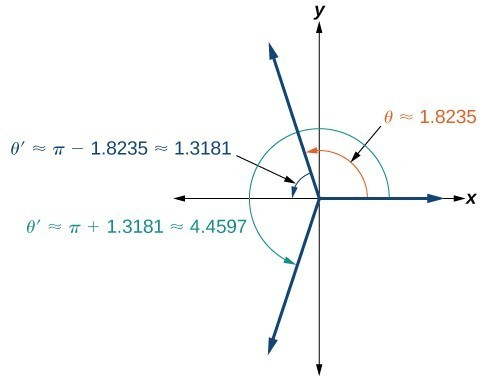So, we also need to find the measure of the angle in quadrant III. In quadrant III, the reference angle is $\theta \text{ }\text{ }\text{‘}\approx \pi -\text{1}\text{.8235}\approx \text{1}\text{.3181}\text{.}$ The other solution in quadrant III is $\theta \text{ }\text{ }\text{‘}\approx \pi +\text{1}\text{.3181}\approx \text{4}\text{.4597}\text{.}$

The solutions are $\theta \approx 1.8235\pm 2\pi k$ and $\theta \approx 4.4597\pm 2\pi k$.

Solve $\cos \theta =-0.2$.

$\theta \approx 1.7722\pm 2\pi k$ and $\theta \approx 4.5110\pm 2\pi k$

## Solving Equations Involving a Single Trigonometric Function

When we are given equations that involve only one of the six trigonometric functions, their solutions involve using algebraic techniques and the unit circle. We need to make several considerations when the equation involves trigonometric functions other than sine and cosine. Problems involving the reciprocals of the primary trigonometric functions need to be viewed from an algebraic perspective. In other words, we will write the reciprocal function, and solve for the angles using the function. Also, an equation involving the tangent function is slightly different from one containing a sine or cosine function. First, as we know, the period of tangent is $\pi$, not $2\pi$. Further, the domain of tangent is all real numbers with the exception of odd integer multiples of $\frac{\pi }{2}$, unless, of course, a problem places its own restrictions on the domain.

## Example 6: Solving a Problem Involving a Single Trigonometric Function

Solve the problem exactly: $2{\sin }^{2}\theta -1=0,0\le \theta <2\pi$.

As this problem is not easily factored, we will solve using the square root property. First, we use algebra to isolate $\sin \theta$. Then we will find the angles.

$\begin{gathered}2{\sin }^{2}\theta -1=0 \\ 2{\sin }^{2}\theta =1 \\ {\sin }^{2}\theta =\frac{1}{2} \\ \sqrt{{\sin }^{2}\theta }=\pm \sqrt{\frac{1}{2}} \\ \sin \theta =\pm \frac{1}{\sqrt{2}}=\pm \frac{\sqrt{2}}{2} \\ \theta =\frac{\pi }{4},\frac{3\pi }{4},\frac{5\pi }{4},\frac{7\pi }{4} \end{gathered}$

## Example 7: Solving a Trigonometric Equation Involving Cosecant

Solve the following equation exactly: $\csc \theta =-2,0\le \theta <4\pi$.

We want all values of $\theta$ for which $\csc \theta =-2$ over the interval $0\le \theta <4\pi$.

$\begin{gathered}\csc \theta =-2 \\ \frac{1}{\sin \theta }=-2 \\ \sin \theta =-\frac{1}{2} \\ \theta =\frac{7\pi }{6},\frac{11\pi }{6},\frac{19\pi }{6},\frac{23\pi }{6} \end{gathered}$

As $\sin \theta =-\frac{1}{2}$, notice that all four solutions are in the third and fourth quadrants.

## Example 8: Solving an Equation Involving Tangent

Solve the equation exactly: $\tan \left(\theta -\frac{\pi }{2}\right)=1,0\le \theta <2\pi$.

Recall that the tangent function has a period of $\pi$. On the interval $\left[0,\pi \right)$, and at the angle of $\frac{\pi }{4}$, the tangent has a value of 1. However, the angle we want is $\left(\theta -\frac{\pi }{2}\right)$. Thus, if $\tan \left(\frac{\pi }{4}\right)=1$, then

$\begin{gathered}\theta -\frac{\pi }{2}=\frac{\pi }{4}\\ \theta =\frac{3\pi }{4}\pm k\pi \end{gathered}$

Over the interval $\left[0,2\pi \right)$, we have two solutions:

$\theta =\frac{3\pi }{4}\text{ and }\theta =\frac{3\pi }{4}+\pi =\frac{7\pi }{4}$

Find all solutions for $\tan x=\sqrt{3}$.

$\frac{\pi }{3}\pm \pi k$

## Example 9: Identify all Solutions to the Equation Involving Tangent

Identify all exact solutions to the equation $2\left(\tan x+3\right)=5+\tan x,0\le x<2\pi$.

We can solve this equation using only algebra. Isolate the expression $\tan x$ on the left side of the equals sign.

$\begin{gathered} 2\left(\tan x\right)+2\left(3\right) =5+\tan x \\ 2\tan x+6 =5+\tan x \\ 2\tan x-\tan x =5 - 6 \\ \tan x =-1\end{gathered}$

There are two angles on the unit circle that have a tangent value of $-1:\theta =\frac{3\pi }{4}$ and $\theta =\frac{7\pi }{4}$.

## Solving Trigonometric Equations in Quadratic Form

Solving a quadratic equation may be more complicated, but once again, we can use algebra as we would for any quadratic equation. Look at the pattern of the equation. Is there more than one trigonometric function in the equation, or is there only one? Which trigonometric function is squared? If there is only one function represented and one of the terms is squared, think about the standard form of a quadratic. Replace the trigonometric function with a variable such as $x$ or $u$. If substitution makes the equation look like a quadratic equation, then we can use the same methods for solving quadratics to solve the trigonometric equations.

## Example 10: Solving a Trigonometric Equation in Quadratic Form

Solve the equation exactly: ${\cos }^{2}\theta +3\cos \theta -1=0,0\le \theta <2\pi$.

We begin by using substitution and replacing cos $\theta$ with $x$. It is not necessary to use substitution, but it may make the problem easier to solve visually. Let $\cos \theta =x$. We have

${x}^{2}+3x - 1=0$

The equation cannot be factored, so we will use the quadratic formula $x=\frac{-b\pm \sqrt{{b}^{2}-4ac}}{2a}$.

\begin{align} x&=\frac{-3\pm \sqrt{{\left(-3\right)}^{2}-4\left(1\right)\left(-1\right)}}{2}&=\frac{-3\pm \sqrt{13}}{2} \end{align}

Replace $x$ with $\cos \theta$, and solve. Thus,

$\begin{gathered} \cos \theta =\frac{-3\pm \sqrt{13}}{2}\theta ={\cos }^{-1}\left(\frac{-3+\sqrt{13}}{2}\right)\end{gathered}$

Note that only the + sign is used. This is because we get an error when we solve $\theta ={\cos }^{-1}\left(\frac{-3-\sqrt{13}}{2}\right)$ on a calculator, since the domain of the inverse cosine function is $\left[-1,1\right]$. However, there is a second solution:

\begin{align}\theta &={\cos }^{-1}\left(\frac{-3+\sqrt{13}}{2}\right) \\ &\approx 1.26 \end{align}

This terminal side of the angle lies in quadrant I. Since cosine is also positive in quadrant IV, the second solution is

\begin{align}\theta &=2\pi -{\cos }^{-1}\left(\frac{-3+\sqrt{13}}{2}\right) \\ &\approx 5.02 \end{align}

## Example 11: Solving a Trigonometric Equation in Quadratic Form by Factoring

Solve the equation exactly: $2{\sin }^{2}\theta -5\sin \theta +3=0,0\le \theta \le 2\pi$.

Using grouping, this quadratic can be factored. Either make the real substitution, $\sin \theta =u$, or imagine it, as we factor:

$\begin{gathered}2{\sin }^{2}\theta -5\sin \theta +3=0 \\ \left(2\sin \theta -3\right)\left(\sin \theta -1\right)=0 \end{gathered}$

Now set each factor equal to zero.

$\begin{gathered}2\sin \theta -3=0 \\ 2\sin \theta =3 \\ \sin \theta =\frac{3}{2} \\ \text{ } \\ \sin \theta -1=0 \\ \sin \theta =1 \end{gathered}$

Next solve for $\theta :\sin \theta \ne \frac{3}{2}$, as the range of the sine function is $\left[-1,1\right]$. However, $\sin \theta =1$, giving the solution $\theta =\frac{\pi }{2}$.

Make sure to check all solutions on the given domain as some factors have no solution.

Solve ${\sin }^{2}\theta =2\cos \theta +2,0\le \theta \le 2\pi$. [Hint: Make a substitution to express the equation only in terms of cosine.]

$\cos \theta =-1,\theta =\pi$

## Example 12: Solving a Trigonometric Equation Using Algebra

Solve exactly:

$2{\sin }^{2}\theta +\sin \theta =0;0\le \theta <2\pi$

This problem should appear familiar as it is similar to a quadratic. Let $\sin \theta =x$. The equation becomes $2{x}^{2}+x=0$. We begin by factoring:

$\begin{gathered}2{x}^{2}+x=0\\ x\left(2x+1\right)=0\end{gathered}$

Set each factor equal to zero.

$\begin{gathered}x=0 \\ 2x+1=0 \\ x=-\frac{1}{2} \end{gathered}$

Then, substitute back into the equation the original expression $\sin \theta$ for $x$. Thus,

$\begin{gathered}\sin \theta =0 \\ \theta =0,\pi \\ \text{ } \\ \sin \theta =-\frac{1}{2} \\ \theta =\frac{7\pi }{6},\frac{11\pi }{6} \end{gathered}$

The solutions within the domain $0\le \theta <2\pi$ are $\theta =0,\pi ,\frac{7\pi }{6},\frac{11\pi }{6}$.

If we prefer not to substitute, we can solve the equation by following the same pattern of factoring and setting each factor equal to zero.

$\begin{gathered}2{\sin }^{2}\theta +\sin \theta =0 \\ \sin \theta \left(2\sin \theta +1\right)=0 \\ \sin \theta =0 \\ \theta =0,\pi \\ \text{ } \\ 2\sin \theta +1=0 \\ 2\sin \theta =-1 \\ \sin \theta =-\frac{1}{2} \\ \theta =\frac{7\pi }{6},\frac{11\pi }{6} \end{gathered}$

We can see the solutions on the graph in Figure 3. On the interval $0\le \theta <2\pi$, the graph crosses the x- axis four times, at the solutions noted. Notice that trigonometric equations that are in quadratic form can yield up to four solutions instead of the expected two that are found with quadratic equations. In this example, each solution (angle) corresponding to a positive sine value will yield two angles that would result in that value.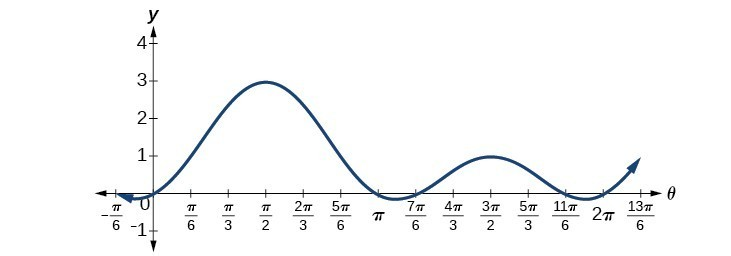We can verify the solutions on the unit circle in Sum and Difference Identities as well.

## Example 13: Solving a Trigonometric Equation Quadratic in Form

Solve the equation quadratic in form exactly: $2{\sin }^{2}\theta -3\sin \theta +1=0,0\le \theta <2\pi$.

We can factor using grouping. Solution values of $\theta$ can be found on the unit circle:

$\begin{gathered}\left(2\sin \theta -1\right)\left(\sin \theta -1\right)=0 \\ 2\sin \theta -1=0 \\ \sin \theta =\frac{1}{2} \\ \theta =\frac{\pi }{6},\frac{5\pi }{6} \\ \text{ } \\ \sin \theta =1 \\ \theta =\frac{\pi }{2} \end{gathered}$

Solve the quadratic equation $2{\cos }^{2}\theta +\cos \theta =0$.

$\frac{\pi }{2},\frac{2\pi }{3},\frac{4\pi }{3},\frac{3\pi }{2}$

## Solving Trigonometric Equations Using Fundamental Identities

While algebra can be used to solve a number of trigonometric equations, we can also use the fundamental identities because they make solving equations simpler. Remember that the techniques we use for solving are not the same as those for verifying identities. The basic rules of algebra apply here, as opposed to rewriting one side of the identity to match the other side. In the next example, we use two identities to simplify the equation.

## Example 14: Use Identities to Solve an Equation

Use identities to solve exactly the trigonometric equation over the interval $0\le x<2\pi$.

$\cos x\cos \left(2x\right)+\sin x\sin \left(2x\right)=\frac{\sqrt{3}}{2}$

Notice that the left side of the equation is the difference formula for cosine.

\begin{align} \cos x\cos \left(2x\right)+\sin x\sin \left(2x\right)&=\frac{\sqrt{3}}{2} \\ \cos \left(x - 2x\right)&=\frac{\sqrt{3}}{2}&& \text{Difference formula for cosine} \\ \cos \left(-x\right)&=\frac{\sqrt{3}}{2}&& \text{Use the negative angle identity}. \\ \cos x&=\frac{\sqrt{3}}{2}\end{align}

From the unit circle in Sum and Difference Identities, we see that $\cos x=\frac{\sqrt{3}}{2}$ when $x=\frac{\pi }{6},\frac{11\pi }{6}$.

## Example 15: Solving the Equation Using a Double-Angle Formula

Solve the equation exactly using a double-angle formula: $\cos \left(2\theta \right)=\cos \theta$.

We have three choices of expressions to substitute for the double-angle of cosine. As it is simpler to solve for one trigonometric function at a time, we will choose the double-angle identity involving only cosine:

$\begin{gathered}\cos \left(2\theta \right)=\cos \theta \\ 2{\cos }^{2}\theta -1=\cos \theta \\ 2{\cos }^{2}\theta -\cos \theta -1=0 \\ \left(2\cos \theta +1\right)\left(\cos \theta -1\right)=0 \\ 2\cos \theta +1=0 \\ \cos \theta =-\frac{1}{2} \\ \text{ } \\ \cos \theta -1=0 \\ \cos \theta =1 \end{gathered}$

So, if $\cos \theta =-\frac{1}{2}$, then $\theta =\frac{2\pi }{3}\pm 2\pi k$ and $\theta =\frac{4\pi }{3}\pm 2\pi k$; if $\cos \theta =1$, then $\theta =0\pm 2\pi k$.

## Example 16: Solving an Equation Using an Identity

Solve the equation exactly using an identity: $3\cos \theta +3=2{\sin }^{2}\theta ,0\le \theta <2\pi$.

If we rewrite the right side, we can write the equation in terms of cosine:

\begin{align} 3 cos\theta +3& ={2 sin}^{2}\theta \\ 3 cos\theta +3& =2\left(1-{\text{cos}}^{2}\theta \right) \\ 3 cos\theta +3& =2 - 2{\cos }^{2}\theta \\ 2{\cos }^{2}\theta +3 cos\theta +1& =0 \\ \left(2 cos\theta +1\right)\left(\cos \theta +1\right)& =0 \\ 2 cos\theta +1& =0 \\ \cos \theta & =-\frac{1}{2} \\ \theta & =\frac{2\pi }{3},\frac{4\pi }{3} \\ \text{ } \\ \cos \theta +1& =0 \\ \cos \theta & =-1 \\ \theta & =\pi\end{align}

Our solutions are $\theta =\frac{2\pi }{3},\frac{4\pi }{3},\pi$.

## Solving Trigonometric Equations with Multiple Angles

Sometimes it is not possible to solve a trigonometric equation with identities that have a multiple angle, such as $\sin \left(2x\right)$ or $\cos \left(3x\right)$. When confronted with these equations, recall that $y=\sin \left(2x\right)$ is a horizontal compression by a factor of 2 of the function $y=\sin x$. On an interval of $2\pi$, we can graph two periods of $y=\sin \left(2x\right)$, as opposed to one cycle of $y=\sin x$. This compression of the graph leads us to believe there may be twice as many x -intercepts or solutions to $\sin \left(2x\right)=0$ compared to $\sin x=0$. This information will help us solve the equation.

## Example 17: Solving a Multiple Angle Trigonometric Equation

Solve exactly: $\cos \left(2x\right)=\frac{1}{2}$ on $\left[0,2\pi \right)$.

We can see that this equation is the standard equation with a multiple of an angle. If $\cos \left(\alpha \right)=\frac{1}{2}$, we know $\alpha$ is in quadrants I and IV. While $\theta ={\cos }^{-1}\frac{1}{2}$ will only yield solutions in quadrants I and II, we recognize that the solutions to the equation $\cos \theta =\frac{1}{2}$ will be in quadrants I and IV.

Therefore, the possible angles are $\theta =\frac{\pi }{3}$ and $\theta =\frac{5\pi }{3}$. So, $2x=\frac{\pi }{3}$ or $2x=\frac{5\pi }{3}$, which means that $x=\frac{\pi }{6}$ or $x=\frac{5\pi }{6}$. Does this make sense? Yes, because $\cos \left(2\left(\frac{\pi }{6}\right)\right)=\cos \left(\frac{\pi }{3}\right)=\frac{1}{2}$.

In quadrant I, $2x=\frac{\pi }{3}$, so $x=\frac{\pi }{6}$ as noted. Let us revolve around the circle again:

\begin{align} 2x&=\frac{\pi }{3}+2\pi \\ &=\frac{\pi }{3}+\frac{6\pi }{3} \\ &=\frac{7\pi }{3} \end{align}

so $x=\frac{7\pi }{6}$.

One more rotation yields

\begin{align} 2x&=\frac{\pi }{3}+4\pi \\ &=\frac{\pi }{3}+\frac{12\pi }{3} \\ &=\frac{13\pi }{3} \end{align}

$x=\frac{13\pi }{6}>2\pi$, so this value for $x$ is larger than $2\pi$, so it is not a solution on $\left[0,2\pi \right)$.

In quadrant IV, $2x=\frac{5\pi }{3}$, so $x=\frac{5\pi }{6}$ as noted. Let us revolve around the circle again:

\begin{align}2x&=\frac{5\pi }{3}+2\pi \\ &=\frac{5\pi }{3}+\frac{6\pi }{3} \\ &=\frac{11\pi }{3}\end{align}

so $x=\frac{11\pi }{6}$.

\begin{align}2x&=\frac{5\pi }{3}+4\pi \\ &=\frac{5\pi }{3}+\frac{12\pi }{3} \\ &=\frac{17\pi }{3} \end{align}

$x=\frac{17\pi }{6}>2\pi$, so this value for $x$ is larger than $2\pi$, so it is not a solution on $\left[0,2\pi \right)$.

Our solutions are $x=\frac{\pi }{6},\frac{5\pi }{6},\frac{7\pi }{6},\text{and }\frac{11\pi }{6}$. Note that whenever we solve a problem in the form of $\sin \left(nx\right)=c$, we must go around the unit circle $n$ times.

## Solving Right Triangle Problems

We can now use all of the methods we have learned to solve problems that involve applying the properties of right triangles and the Pythagorean Theorem . We begin with the familiar Pythagorean Theorem, ${a}^{2}+{b}^{2}={c}^{2}$, and model an equation to fit a situation.

## Example 18: Using the Pythagorean Theorem to Model an Equation

Use the Pythagorean Theorem, and the properties of right triangles to model an equation that fits the problem.

One of the cables that anchors the center of the London Eye Ferris wheel to the ground must be replaced. The center of the Ferris wheel is 69.5 meters above the ground, and the second anchor on the ground is 23 meters from the base of the Ferris wheel. Approximately how long is the cable, and what is the angle of elevation (from ground up to the center of the Ferris wheel)?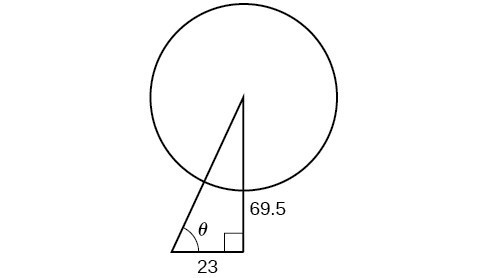Using the information given, we can draw a right triangle. We can find the length of the cable with the Pythagorean Theorem.

$\begin{gathered}{a}^{2}+{b}^{2}={c}^{2} \\ {\left(23\right)}^{2}+{\left(69.5\right)}^{2}\approx 5359 \\ \sqrt{5359}\approx 73.2\text{ m}\end{gathered}$

The angle of elevation is $\theta$, formed by the second anchor on the ground and the cable reaching to the center of the wheel. We can use the tangent function to find its measure. Round to two decimal places.

\begin{align}\tan \theta &=\frac{69.5}{23} \\ {\tan }^{-1}\left(\frac{69.5}{23}\right)&\approx 1.2522 \\ &\approx {71.69}^{\circ } \end{align}

The angle of elevation is approximately ${71.7}^{\circ }$, and the length of the cable is 73.2 meters.

## Example 19: Using the Pythagorean Theorem to Model an Abstract Problem

OSHA safety regulations require that the base of a ladder be placed 1 foot from the wall for every 4 feet of ladder length. Find the angle that a ladder of any length forms with the ground and the height at which the ladder touches the wall.

For any length of ladder, the base needs to be a distance from the wall equal to one fourth of the ladder’s length. Equivalently, if the base of the ladder is “ a” feet from the wall, the length of the ladder will be 4 a feet.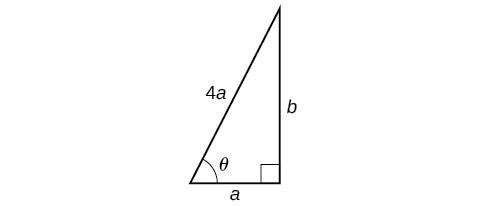The side adjacent to $\theta$ is a and the hypotenuse is $4a$. Thus,

$\begin{gathered}\cos \theta =\frac{a}{4a}=\frac{1}{4} \\ {\cos }^{-1}\left(\frac{1}{4}\right)\approx {75.5}^{\circ } \end{gathered}$

The elevation of the ladder forms an angle of ${75.5}^{\circ }$ with the ground. The height at which the ladder touches the wall can be found using the Pythagorean Theorem:

$\begin{gathered}{a}^{2}+{b}^{2}={\left(4a\right)}^{2} \\ {b}^{2}={\left(4a\right)}^{2}-{a}^{2} \\ {b}^{2}=16{a}^{2}-{a}^{2} \\ {b}^{2}=15{a}^{2} \\ b=\sqrt{15}a \end{gathered}$

Thus, the ladder touches the wall at $\sqrt{15}a$ feet from the ground.

## Key Concepts

• When solving linear trigonometric equations, we can use algebraic techniques just as we do solving algebraic equations. Look for patterns, like the difference of squares, quadratic form, or an expression that lends itself well to substitution.
• Equations involving a single trigonometric function can be solved or verified using the unit circle.
• We can also solve trigonometric equations using a graphing calculator.
• Many equations appear quadratic in form. We can use substitution to make the equation appear simpler, and then use the same techniques we use solving an algebraic quadratic: factoring, the quadratic formula, etc.
• We can also use the identities to solve trigonometric equation.
• We can use substitution to solve a multiple-angle trigonometric equation, which is a compression of a standard trigonometric function. We will need to take the compression into account and verify that we have found all solutions on the given interval.
• Real-world scenarios can be modeled and solved using the Pythagorean Theorem and trigonometric functions.
• Precalculus. Authored by : OpenStax College. Provided by : OpenStax. Located at : http://cnx.org/contents/fd53eae1-fa23-47c7-[email protected]:1/Preface . License : CC BY: Attribution
• EXPLORE Tech Help Pro About Us Random Article Quizzes Request a New Article Community Dashboard This Or That Game Popular Categories Arts and Entertainment Artwork Books Movies Computers and Electronics Computers Phone Skills Technology Hacks Health Men's Health Mental Health Women's Health Relationships Dating Love Relationship Issues Hobbies and Crafts Crafts Drawing Games Education & Communication Communication Skills Personal Development Studying Personal Care and Style Fashion Hair Care Personal Hygiene Youth Personal Care School Stuff Dating All Categories Arts and Entertainment Finance and Business Home and Garden Relationship Quizzes Cars & Other Vehicles Food and Entertaining Personal Care and Style Sports and Fitness Computers and Electronics Health Pets and Animals Travel Education & Communication Hobbies and Crafts Philosophy and Religion Work World Family Life Holidays and Traditions Relationships Youth
• Browse Articles
• Learn Something New
• Quizzes Hot
• This Or That Game New
• Explore More
• Support wikiHow
• Education and Communications
• Mathematics
• Trigonometry

## How to Solve Trigonometric Equations

Last Updated: February 1, 2023 Fact Checked

wikiHow is a “wiki,” similar to Wikipedia, which means that many of our articles are co-written by multiple authors. To create this article, 17 people, some anonymous, worked to edit and improve it over time. There are 10 references cited in this article, which can be found at the bottom of the page. This article has been fact-checked, ensuring the accuracy of any cited facts and confirming the authority of its sources. This article has been viewed 253,069 times. Learn more...

Did you get homework from your teacher that was about solving Trigonometric equations? Did you maybe not pay full attention in class during the lesson on Trigonometric questions? Do you even know what "Trigonometric" means? If you answered yes to these questions, then you don't need to worry because this wikiHow will teach you how to solve Trigonometric equations.• To solve a trig equation, transform it into one or many basic trig equations. Solving trig equations finally results in solving 4 types of basic trig equations.• There are 4 types of basic trig equations:
• sin x = a ; cos x = a
• tan x = a ; cot x = a
• Solving basic trig equations proceeds by studying the various positions of the arc x on the trig circle, and by using trig conversion table (or calculator ). To fully know how to solve these basic trig equations, and similar, see book titled :"Trigonometry: Solving trig equations and inequalities" (Amazon E-book 2010).
• Example 1. Solve sin x = 0.866. The conversion table (or calculator) gives the answer: x = Pi/3. The trig circle gives another arc (2Pi/3) that has the same sin value (0.866). The trig circle also gives an infinity of answers that are called extended answers.
• x1 = Pi/3 + 2k.Pi, and x2 = 2Pi/3. (Answers within period (0, 2Pi))
• x1 = Pi/3 + 2k Pi, and x2 = 2Pi/3 + 2k Pi. (Extended answers).
• Example 2. Solve: cos x = -1/2. Calculators give x = 2 Pi/3. The trig circle gives another x = -2Pi/3.
• x1 = 2Pi/3 + 2k.Pi, and x2 = - 2Pi/3. (Answers within period (0, 2Pi))
• x1 = 2Pi/3 + 2k Pi, and x2 = -2Pi/3 + 2k.Pi. (Extended answers)
• Example 3. Solve: tan (x - Pi/4) = 0.
• x = Pi/4 ; (Answer)
• x = Pi/4 + k Pi; ( Extended answer)
• Example 4. Solve cot 2x = 1.732. Calculators and the trig circle give
• x = Pi/12 ; (Answer)
• x = Pi/12 + k Pi ; (Extended answers)• To transform a given trig equation into basic trig ones, use common algebraic transformations ( factoring , common factor , polynomial identities...), definitions and properties of trig functions, and trig identities. There are about 31, among them the last 14 trig identities, from 19 to 31, are called Transformation Identities, since they are used in the transformation of trig equations.  X Research source See book mentioned above.
• Example 5: The trig equation: sin x + sin 2x + sin 3x = 0 can be transformed, using trig identities, into a product of basic trig equations: 4cos x*sin (3x/2)*cos (x/2) = 0. The basic trig equations to be solved are: cos x = 0 ; sin (3x/2) = 0 ; and cos (x/2) = 0.• Before learning solving trig equations, you must know how to quickly find the arcs whose trig functions are known. Conversion values of arcs (or angles) are given by trig tables or calculators.  X Research source
• Example: After solving, get cos x = 0.732. Calculators give the solution arc x = 42.95 degree. The trig unit circle will give other solution arcs that have the same cos value.• You can graph to illustrate the solution arcs on the trig unit circle. The terminal points of these solution arcs constitute regular polygons on the trig circle. For examples:
• The terminal points of the solution arcs x = Pi/3 + k.Pi/2 constitute a square on the trig unit circle.
• The solution arcs x = Pi/4 + k.Pi/3 are represented by the vertexes of a regular hexagon on the trig unit circle.• A. Approach 1.
• Transform the given trig equation into a product in the form: f(x).g(x) = 0 or f(x).g(x).h(x) = 0, in which f(x), g(x) and h(x) are basic trig equations.
• Example 6. Solve: 2cos x + sin 2x = 0. (0 < x < 2Pi)
• Solution. Replace in the equation sin 2x by using the identity: sin 2x = 2*sin x*cos x.
• cos x + 2*sin x*cos x = 2cos x*( sin x + 1) = 0. Next, solve the 2 basic trig functions: cos x = 0, and (sin x + 1) = 0.
• Example 7. Solve: cos x + cos 2x + cos 3x = 0. (0 < x < 2Pi)
• Solution: Transform it to a product, using trig identities: cos 2x(2cos x + 1 ) = 0. Next, solve the 2 basic trig equations: cos 2x = 0, and (2cos x + 1) = 0.
• Example 8. Solve: sin x - sin 3x = cos 2x. (0 < x < 2Pi)
• B. Approach 2.
• Transform the given trig equation into a trig equation having only one unique trig function as variable. There are a few tips on how to select the appropriate variable. The common variables to select are: sin x = t; cos x = t; cos 2x = t, tan x = t and tan (x/2) = t.
• Example 9. Solve: 3sin^2 x - 2cos^2 x = 4sin x + 7 (0 < x < 2Pi).
• Solution. Replace in the equation (cos^2 x) by (1 - sin^2 x), then simplify the equation:
• 3sin^2 x - 2 + 2sin^2 x - 4sin x - 7 = 0. Call sin x = t. The equation becomes: 5t^2 - 4t - 9 = 0. This is a quadratic equation that has 2 real roots: t1 = -1 and t2 = 9/5. The second t2 is rejected since > 1. Next, solve: t = sin = -1 --> x = 3Pi/2.
• Example 10. Solve: tan x + 2 tan^2 x = cot x + 2.
• Solution. Call tan x = t. Transform the given equation into an equation with t as variable: (2t + 1)(t^2 - 1) = 0. Solve for t from this product, then solve the basic trig equation tan x = t for x.• There are a few special types of trig equations that require some specific transformations. Examples:
• a*sin x+ b*cos x = c ; a(sin x + cos x) + b*cos x*sin x = c ;
• a*sin^2 x + b*sin x*cos x + c*cos^2 x = 0• The function f(x) = sin x has 2Pi as period.
• The function f(x) = tan x has Pi as period.
• The function f(x) = sin 2x has Pi as period.
• The function f(x) = cos (x/2) has 4Pi as period.
• If the period is specified in the problem/test, you have to only find the solution arc(s) x within this period.
• NOTE: Solving trig equation is a tricky work that often leads to errors and mistakes. Therefore, answers should be carefully checked. After solving, you can check the answers by using a graphing calculator to directly graph the given trig equation R(x) = 0. The answers (real roots) will be given in decimals. For example, Pi is given by the value 3.14
• ↑ https://www.purplemath.com/modules/solvtrig.htm
• ↑ https://www.mathopenref.com/arcsin.html
• ↑ https://courses.lumenlearning.com/precalculus/chapter/unit-circle-sine-and-cosine-functions/
• ↑ https://mathbitsnotebook.com/Algebra2/TrigConcepts/TCEquationsMore.html
• ↑ https://www.analyzemath.com/trigonometry/properties.html

## Community Q&A## You Might Also Like• Send fan mail to authors## Featured Articles## Trending Articles## Watch Articles• Do Not Sell or Share My Info
• Not Selling Info

## Introduction to Trigonometry

Trigonometry (from Greek trigonon "triangle" + metron "measure")

Want to learn Trigonometry? Here is a quick summary. Follow the links for more, or go to Trigonometry Index

Trigonometry helps us find angles and distances, and is used a lot in science, engineering, video games, and more!

## Right-Angled Triangle

The triangle of most interest is the right-angled triangle . The right angle is shown by the little box in the corner:

Another angle is often labeled θ , and the three sides are then called:

• Opposite : opposite the angle θ
• and the longest side is the Hypotenuse

## Why a Right-Angled Triangle?

Why is this triangle so important?

Imagine we can measure along and up but want to know the direct distance and angle:

Trigonometry can find that missing angle and distance.

Or maybe we have a distance and angle and need to "plot the dot" along and up:

Questions like these are common in engineering, computer animation and more.

## Sine, Cosine and Tangent

The main functions in trigonometry are Sine, Cosine and Tangent

They are simply one side of a right-angled triangle divided by another.

For any angle " θ ":

(Sine, Cosine and Tangent are often abbreviated to sin, cos and tan .)## Example: What is the sine of 35°?

Using this triangle (lengths are only to one decimal place):

sin(35°) = Opposite Hypotenuse = 2.8 4.9 = 0.57...

The triangle could be larger, smaller or turned around, but that angle will always have that ratio .

Calculators have sin, cos and tan to help us, so let's see how to use them:

## Example: How Tall is The Tree?

We can't reach the top of the tree, so we walk away and measure an angle (using a protractor) and distance (using a laser):

• We know the Hypotenuse
• And we want to know the Opposite

Sine is the ratio of Opposite / Hypotenuse :

sin(45°) = Opposite HypotenuseGet a calculator, type in "45", then the "sin" key:

sin(45°) = 0.7071...

What does the 0.7071... mean? It is the ratio of the side lengths, so the Opposite is about 0.7071 times as long as the Hypotenuse.

We can now put 0.7071... in place of sin(45°):

0.7071... = Opposite Hypotenuse

And we also know the hypotenuse is 20 :

0.7071... = Opposite 20

To solve, first multiply both sides by 20:

20 × 0.7071... = Opposite

Opposite = 14.14m (to 2 decimals)

The tree is 14.14m tall

## Try Sin Cos and Tan

Play with this for a while (move the mouse around) and get familiar with values of sine, cosine and tangent for different angles, such as 0°, 30°, 45°, 60° and 90°.

Also try 120°, 135°, 180°, 240°, 270° etc, and notice that positions can be positive or negative by the rules of Cartesian coordinates , so the sine, cosine and tangent change between positive and negative also.

So trigonometry is also about circles !

## Unit Circle

What you just played with is the Unit Circle .

It is a circle with a radius of 1 with its center at 0.

Because the radius is 1, we can directly measure sine, cosine and tangent.

Here we see the sine function being made by the unit circle:

Note: you can see the nice graphs made by sine, cosine and tangent .

Angles can be in Degrees or Radians . Here are some examples:

## Repeating Pattern

Because the angle is rotating around and around the circle the Sine, Cosine and Tangent functions repeat once every full rotation (see Amplitude, Period, Phase Shift and Frequency ).

When we want to calculate the function for an angle larger than a full rotation of 360° (2 π radians) we subtract as many full rotations as needed to bring it back below 360° (2 π radians):

## Example: what is the cosine of 370°?

370° is greater than 360° so let us subtract 360°

370° − 360° = 10°

cos(370°) = cos(10°) = 0.985 (to 3 decimal places)

And when the angle is less than zero, just add full rotations.

## Example: what is the sine of −3 radians?

−3 is less than 0 so let us add 2 π radians

−3 + 2 π = −3 + 6.283... = 3.283... rad ians

sin(−3) = sin(3.283...) = −0.141 (to 3 decimal places)

## Solving Triangles

Trigonometry is also useful for general triangles, not just right-angled ones .

It helps us in Solving Triangles . "Solving" means finding missing sides and angles.

## Example: Find the Missing Angle "C"

Angle C can be found using angles of a triangle add to 180° :

So C = 180° − 76° − 34° = 70°

We can also find missing side lengths. The general rule is:

When we know any 3 of the sides or angles we can find the other 3 (except for the three angles case)

See Solving Triangles for more details.

## Other Functions (Cotangent, Secant, Cosecant)

Similar to Sine, Cosine and Tangent, there are three other trigonometric functions which are made by dividing one side by another:

## Trigonometric and Triangle Identities

And as you get better at Trigonometry you can learn these:

Enjoy becoming a triangle (and circle) expert!

• Varsity Tutors
• K-5 Subjects
• Study Skills
• All AP Subjects
• AP Calculus
• AP Chemistry
• AP Computer Science
• AP Human Geography
• AP Macroeconomics
• AP Microeconomics
• AP Statistics
• AP US History
• AP World History
• Microsoft Excel
• Supply Chain Management
• All Humanities
• Essay Editing
• All Languages
• Mandarin Chinese
• Portuguese Chinese
• Sign Language
• All Learning Differences
• Learning Disabilities
• Special Education
• College Math
• Common Core Math
• Elementary School Math
• High School Math
• Middle School Math
• Pre-Calculus
• Trigonometry
• All Science
• Organic Chemistry
• Physical Chemistry
• All Engineering
• Chemical Engineering
• Civil Engineering
• Computer Science
• Electrical Engineering
• Industrial Engineering
• Materials Science & Engineering
• Mechanical Engineering
• Thermodynamics
• Biostatistics
• College Essays
• High School
• 1-on-1 Private Tutoring
• Online Tutoring
• Instant Tutoring
• Pricing Info
• All AP Exams
• ACT Tutoring
• ACT Science
• ACT Writing
• SAT Tutoring
• SAT Writing
• GRE Tutoring
• NCLEX Tutoring
• And more...
• StarCourses
• Beginners Coding
• Early Childhood
• For Schools Overview
• Talk with Our Team
• Reviews & Testimonials
• Press & Media Coverage
• Tutor/Instructor Jobs
• Corporate Solutions
• Become a Tutor

## Solving Trigonometric Equations using Algebraic Methods

An equation that contains trigonometric functions is called a trigonometric equation .

sin 2 x + cos 2 x = 1 2 sin x − 1 = 0 tan 2 2 x − 1 = 0

## Solving Trigonometric Equations

To solve a trigonometric equation, we use the rules of algebra to isolate the trigonometric function on one side of the equal sign. Then we use our knowledge of the values of the trigonometric functions to solve for the variable.

When you solve a trigonometric equation that involves only one trigonometric expression, begin by isolating the expression.

When trigonometric functions cannot be combined on one side of an equation, try to factor the equation and then apply zero product property to solve the equation. If the equation has quadratic form, first factor if possible. If not possible, apply the quadratic formula to solve the equation.

Solve 2 sin x − 1 = 0 .

To solve the equation, we begin by rewriting it so that sin x is isolated on the left side. So, first add 1 to each side and then divide each side by 2 .

2 sin x = 1 sin x = 1 2

Since sin x has a period of 2 π , first we find all the solutions in the interval [ 0 , 2 π ] .

The solutions are x = π 6 and x = 5 π 6 .

The solutions on the interval ( − ∞ , ∞ ) are then found by adding integer multiples of 2 π . Adding 2 n π to each of the solutions, we obtain the general solution of the given equation. Therefore, the general form of the solutions is x = π 6 + 2 n π and x = 5 π 6 + 2 n π where n is any integer.• 7.1 Solving Trigonometric Equations with Identities
• Introduction to Functions
• 1.1 Functions and Function Notation
• 1.2 Domain and Range
• 1.3 Rates of Change and Behavior of Graphs
• 1.4 Composition of Functions
• 1.5 Transformation of Functions
• 1.6 Absolute Value Functions
• 1.7 Inverse Functions
• Key Equations
• Key Concepts
• Review Exercises
• Practice Test
• Introduction to Linear Functions
• 2.1 Linear Functions
• 2.2 Graphs of Linear Functions
• 2.3 Modeling with Linear Functions
• 2.4 Fitting Linear Models to Data
• Introduction to Polynomial and Rational Functions
• 3.1 Complex Numbers
• 3.3 Power Functions and Polynomial Functions
• 3.4 Graphs of Polynomial Functions
• 3.5 Dividing Polynomials
• 3.6 Zeros of Polynomial Functions
• 3.7 Rational Functions
• 3.8 Inverses and Radical Functions
• 3.9 Modeling Using Variation
• Introduction to Exponential and Logarithmic Functions
• 4.1 Exponential Functions
• 4.2 Graphs of Exponential Functions
• 4.3 Logarithmic Functions
• 4.4 Graphs of Logarithmic Functions
• 4.5 Logarithmic Properties
• 4.6 Exponential and Logarithmic Equations
• 4.7 Exponential and Logarithmic Models
• 4.8 Fitting Exponential Models to Data
• Introduction to Trigonometric Functions
• 5.2 Unit Circle: Sine and Cosine Functions
• 5.3 The Other Trigonometric Functions
• 5.4 Right Triangle Trigonometry
• Introduction to Periodic Functions
• 6.1 Graphs of the Sine and Cosine Functions
• 6.2 Graphs of the Other Trigonometric Functions
• 6.3 Inverse Trigonometric Functions
• Introduction to Trigonometric Identities and Equations
• 7.2 Sum and Difference Identities
• 7.3 Double-Angle, Half-Angle, and Reduction Formulas
• 7.4 Sum-to-Product and Product-to-Sum Formulas
• 7.5 Solving Trigonometric Equations
• 7.6 Modeling with Trigonometric Functions
• Introduction to Further Applications of Trigonometry
• 8.1 Non-right Triangles: Law of Sines
• 8.2 Non-right Triangles: Law of Cosines
• 8.3 Polar Coordinates
• 8.4 Polar Coordinates: Graphs
• 8.5 Polar Form of Complex Numbers
• 8.6 Parametric Equations
• 8.7 Parametric Equations: Graphs
• 8.8 Vectors
• Introduction to Systems of Equations and Inequalities
• 9.1 Systems of Linear Equations: Two Variables
• 9.2 Systems of Linear Equations: Three Variables
• 9.3 Systems of Nonlinear Equations and Inequalities: Two Variables
• 9.4 Partial Fractions
• 9.5 Matrices and Matrix Operations
• 9.6 Solving Systems with Gaussian Elimination
• 9.7 Solving Systems with Inverses
• 9.8 Solving Systems with Cramer's Rule
• Introduction to Analytic Geometry
• 10.1 The Ellipse
• 10.2 The Hyperbola
• 10.3 The Parabola
• 10.4 Rotation of Axes
• 10.5 Conic Sections in Polar Coordinates
• Introduction to Sequences, Probability and Counting Theory
• 11.1 Sequences and Their Notations
• 11.2 Arithmetic Sequences
• 11.3 Geometric Sequences
• 11.4 Series and Their Notations
• 11.5 Counting Principles
• 11.6 Binomial Theorem
• 11.7 Probability
• Introduction to Calculus
• 12.1 Finding Limits: Numerical and Graphical Approaches
• 12.2 Finding Limits: Properties of Limits
• 12.3 Continuity
• 12.4 Derivatives
• A | Basic Functions and Identities

## Learning Objectives

In this section, you will:

• Verify the fundamental trigonometric identities.
• Simplify trigonometric expressions using algebra and the identities.In espionage movies, we see international spies with multiple passports, each claiming a different identity. However, we know that each of those passports represents the same person. The trigonometric identities act in a similar manner to multiple passports—there are many ways to represent the same trigonometric expression. Just as a spy will choose an Italian passport when traveling to Italy, we choose the identity that applies to the given scenario when solving a trigonometric equation.

In this section, we will begin an examination of the fundamental trigonometric identities, including how we can verify them and how we can use them to simplify trigonometric expressions.

## Verifying the Fundamental Trigonometric Identities

Identities enable us to simplify complicated expressions. They are the basic tools of trigonometry used in solving trigonometric equations, just as factoring, finding common denominators, and using special formulas are the basic tools of solving algebraic equations. In fact, we use algebraic techniques constantly to simplify trigonometric expressions. Basic properties and formulas of algebra, such as the difference of squares formula and the perfect squares formula, will simplify the work involved with trigonometric expressions and equations. We already know that all of the trigonometric functions are related because they all are defined in terms of the unit circle. Consequently, any trigonometric identity can be written in many ways.

To verify the trigonometric identities, we usually start with the more complicated side of the equation and essentially rewrite the expression until it has been transformed into the same expression as the other side of the equation. Sometimes we have to factor expressions, expand expressions, find common denominators, or use other algebraic strategies to obtain the desired result. In this first section, we will work with the fundamental identities: the Pythagorean Identities , the even-odd identities, the reciprocal identities, and the quotient identities.

We will begin with the Pythagorean Identities (see Table 1 ), which are equations involving trigonometric functions based on the properties of a right triangle. We have already seen and used the first of these identifies, but now we will also use additional identities.

The second and third identities can be obtained by manipulating the first. The identity 1 + cot 2 θ = csc 2 θ 1 + cot 2 θ = csc 2 θ is found by rewriting the left side of the equation in terms of sine and cosine.

Prove: 1 + cot 2 θ = csc 2 θ 1 + cot 2 θ = csc 2 θ

Similarly, 1 + tan 2 θ = sec 2 θ 1 + tan 2 θ = sec 2 θ can be obtained by rewriting the left side of this identity in terms of sine and cosine. This gives

The next set of fundamental identities is the set of even-odd identities. The even-odd identities relate the value of a trigonometric function at a given angle to the value of the function at the opposite angle and determine whether the identity is odd or even. (See Table 2 ).

Recall that an odd function is one in which f (− x ) = − f ( x ) f (− x ) = − f ( x ) for all x x in the domain of f . f . The sine function is an odd function because sin ( − θ ) = − sin θ . sin ( − θ ) = − sin θ . The graph of an odd function is symmetric about the origin. For example, consider corresponding inputs of π 2 π 2 and − π 2 . − π 2 . The output of sin ( π 2 ) sin ( π 2 ) is opposite the output of sin ( − π 2 ) . sin ( − π 2 ) . Thus,

This is shown in Figure 2 .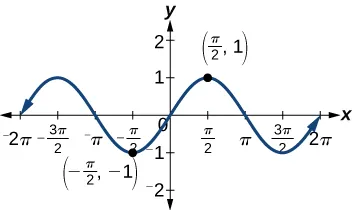Recall that an even function is one in which

The graph of an even function is symmetric about the y- axis. The cosine function is an even function because cos ( − θ ) = cos θ . cos ( − θ ) = cos θ . For example, consider corresponding inputs π 4 π 4 and − π 4 . − π 4 . The output of cos ( π 4 ) cos ( π 4 ) is the same as the output of cos ( − π 4 ) . cos ( − π 4 ) . Thus,

See Figure 3 .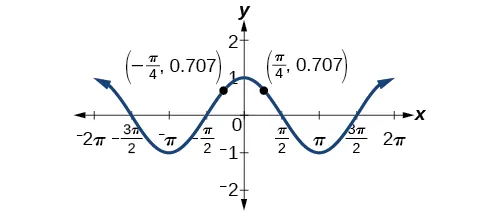For all θ θ in the domain of the sine and cosine functions, respectively, we can state the following:

• Since sin (− θ ) = − sin θ , sin (− θ ) = − sin θ , sine is an odd function.
• Since, cos (− θ ) = cos θ , cos (− θ ) = cos θ , cosine is an even function.

The other even-odd identities follow from the even and odd nature of the sine and cosine functions. For example, consider the tangent identity, tan (− θ ) = −tan θ . tan (− θ ) = −tan θ . We can interpret the tangent of a negative angle as tan (− θ ) = sin ( − θ ) cos (− θ ) = − sin θ cos θ = − tan θ . tan (− θ ) = sin ( − θ ) cos (− θ ) = − sin θ cos θ = − tan θ . Tangent is therefore an odd function, which means that tan ( − θ ) = − tan ( θ ) tan ( − θ ) = − tan ( θ ) for all θ θ in the domain of the tangent function .

The cotangent identity, cot ( − θ ) = − cot θ , cot ( − θ ) = − cot θ , also follows from the sine and cosine identities. We can interpret the cotangent of a negative angle as cot ( − θ ) = cos ( − θ ) sin ( − θ ) = cos θ − sin θ = − cot θ . cot ( − θ ) = cos ( − θ ) sin ( − θ ) = cos θ − sin θ = − cot θ . Cotangent is therefore an odd function, which means that cot ( − θ ) = − cot ( θ ) cot ( − θ ) = − cot ( θ ) for all θ θ in the domain of the cotangent function .

The cosecant function is the reciprocal of the sine function, which means that the cosecant of a negative angle will be interpreted as csc ( − θ ) = 1 sin ( − θ ) = 1 − sin θ = − csc θ . csc ( − θ ) = 1 sin ( − θ ) = 1 − sin θ = − csc θ . The cosecant function is therefore odd.

Finally, the secant function is the reciprocal of the cosine function, and the secant of a negative angle is interpreted as sec ( − θ ) = 1 cos ( − θ ) = 1 cos θ = sec θ . sec ( − θ ) = 1 cos ( − θ ) = 1 cos θ = sec θ . The secant function is therefore even.

To sum up, only two of the trigonometric functions, cosine and secant, are even. The other four functions are odd, verifying the even-odd identities.

The next set of fundamental identities is the set of reciprocal identities , which, as their name implies, relate trigonometric functions that are reciprocals of each other. See Table 3 .

The final set of identities is the set of quotient identities , which define relationships among certain trigonometric functions and can be very helpful in verifying other identities. See Table 4 .

The reciprocal and quotient identities are derived from the definitions of the basic trigonometric functions.

## Summarizing Trigonometric Identities

The Pythagorean Identities are based on the properties of a right triangle.

The even-odd identities relate the value of a trigonometric function at a given angle to the value of the function at the opposite angle.

The reciprocal identities define reciprocals of the trigonometric functions.

The quotient identities define the relationship among the trigonometric functions.

## Graphing the Equations of an Identity

Graph both sides of the identity cot θ = 1 tan θ . cot θ = 1 tan θ . In other words, on the graphing calculator, graph y = cot θ y = cot θ and y = 1 tan θ . y = 1 tan θ .

See Figure 4 .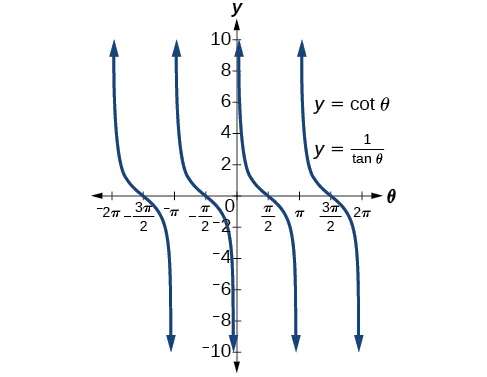We see only one graph because both expressions generate the same image. One is on top of the other. This is a good way to confirm an identity verified with analytical means. If both expressions give the same graph, then they are most likely identities.

Given a trigonometric identity, verify that it is true.

• Work on one side of the equation. It is usually better to start with the more complex side, as it is easier to simplify than to build.
• Look for opportunities to factor expressions, square a binomial, or add fractions.
• Noting which functions are in the final expression, look for opportunities to use the identities and make the proper substitutions.
• If these steps do not yield the desired result, try converting all terms to sines and cosines.

## Verifying a Trigonometric Identity

Verify tan θ cos θ = sin θ . tan θ cos θ = sin θ .

We will start on the left side, as it is the more complicated side:

This identity was fairly simple to verify, as it only required writing tan θ tan θ in terms of sin θ sin θ and cos θ . cos θ .

Verify the identity csc θ cos θ tan θ = 1. csc θ cos θ tan θ = 1.

## Verifying the Equivalency Using the Even-Odd Identities

Verify the following equivalency using the even-odd identities:

Working on the left side of the equation, we have

## Verifying a Trigonometric Identity Involving sec 2 θ

Verify the identity sec 2 θ − 1 sec 2 θ = sin 2 θ sec 2 θ − 1 sec 2 θ = sin 2 θ

As the left side is more complicated, let’s begin there.

There is more than one way to verify an identity. Here is another possibility. Again, we can start with the left side.

In the first method, we used the identity sec 2 θ = tan 2 θ + 1 sec 2 θ = tan 2 θ + 1 and continued to simplify. In the second method, we split the fraction, putting both terms in the numerator over the common denominator. This problem illustrates that there are multiple ways we can verify an identity. Employing some creativity can sometimes simplify a procedure. As long as the substitutions are correct, the answer will be the same.

Show that cot θ csc θ = cos θ . cot θ csc θ = cos θ .

## Creating and Verifying an Identity

Create an identity for the expression 2 tan θ sec θ 2 tan θ sec θ by rewriting strictly in terms of sine.

There are a number of ways to begin, but here we will use the quotient and reciprocal identities to rewrite the expression:

## Verifying an Identity Using Algebra and Even/Odd Identities

Verify the identity:

Verify the identity sin 2 θ − 1 tan θ sin θ − tan θ = sin θ + 1 tan θ . sin 2 θ − 1 tan θ sin θ − tan θ = sin θ + 1 tan θ .

## Verifying an Identity Involving Cosines and Cotangents

Verify the identity: ( 1 − cos 2 x ) ( 1 + cot 2 x ) = 1. ( 1 − cos 2 x ) ( 1 + cot 2 x ) = 1.

We will work on the left side of the equation.

## Using Algebra to Simplify Trigonometric Expressions

We have seen that algebra is very important in verifying trigonometric identities, but it is just as critical in simplifying trigonometric expressions before solving. Being familiar with the basic properties and formulas of algebra, such as the difference of squares formula, the perfect square formula, or substitution, will simplify the work involved with trigonometric expressions and equations.

For example, the equation ( sin x + 1 ) ( sin x − 1 ) = 0 ( sin x + 1 ) ( sin x − 1 ) = 0 resembles the equation ( x + 1 ) ( x − 1 ) = 0 , ( x + 1 ) ( x − 1 ) = 0 , which uses the factored form of the difference of squares. Using algebra makes finding a solution straightforward and familiar. We can set each factor equal to zero and solve. This is one example of recognizing algebraic patterns in trigonometric expressions or equations.

Another example is the difference of squares formula, a 2 − b 2 = ( a − b ) ( a + b ) , a 2 − b 2 = ( a − b ) ( a + b ) , which is widely used in many areas other than mathematics, such as engineering, architecture, and physics. We can also create our own identities by continually expanding an expression and making the appropriate substitutions. Using algebraic properties and formulas makes many trigonometric equations easier to understand and solve.

## Writing the Trigonometric Expression as an Algebraic Expression

Write the following trigonometric expression as an algebraic expression: 2 cos 2 θ + cos θ − 1. 2 cos 2 θ + cos θ − 1.

Notice that the pattern displayed has the same form as a standard quadratic expression, a x 2 + b x + c . a x 2 + b x + c . Letting cos θ = x , cos θ = x , we can rewrite the expression as follows:

This expression can be factored as ( 2 x − 1 ) ( x + 1 ) . ( 2 x − 1 ) ( x + 1 ) . If it were set equal to zero and we wanted to solve the equation, we would use the zero factor property and solve each factor for x . x . At this point, we would replace x x with cos θ cos θ and solve for θ . θ .

## Rewriting a Trigonometric Expression Using the Difference of Squares

Rewrite the trigonometric expression: 4 cos 2 θ − 1. 4 cos 2 θ − 1.

Notice that both the coefficient and the trigonometric expression in the first term are squared, and the square of the number 1 is 1. This is the difference of squares. Thus,

If this expression were written in the form of an equation set equal to zero, we could solve each factor using the zero factor property. We could also use substitution like we did in the previous problem and let cos θ = x , cos θ = x , rewrite the expression as 4 x 2 − 1 , 4 x 2 − 1 , and factor ( 2 x − 1 ) ( 2 x + 1 ) . ( 2 x − 1 ) ( 2 x + 1 ) . Then replace x x with cos θ cos θ and solve for the angle.

Rewrite the trigonometric expression: 25 − 9 sin 2 θ . 25 − 9 sin 2 θ .

## Simplify by Rewriting and Using Substitution

Simplify the expression by rewriting and using identities:

Now we can simplify by substituting 1 + cot 2 θ 1 + cot 2 θ for csc 2 θ . csc 2 θ . We have

Use algebraic techniques to verify the identity: cos θ 1 + sin θ = 1 − sin θ cos θ . cos θ 1 + sin θ = 1 − sin θ cos θ .

(Hint: Multiply the numerator and denominator on the left side by 1 − sin θ . ) 1 − sin θ . )

Access these online resources for additional instruction and practice with the fundamental trigonometric identities.

• Fundamental Trigonometric Identities
• Verifying Trigonometric Identities

## 7.1 Section Exercises

We know g ( x ) = cos x g ( x ) = cos x is an even function, and f ( x ) = sin x f ( x ) = sin x and h ( x ) = tan x h ( x ) = tan x are odd functions. What about G ( x ) = cos 2 x , F ( x ) = sin 2 x , G ( x ) = cos 2 x , F ( x ) = sin 2 x , and H ( x ) = tan 2 x ? H ( x ) = tan 2 x ? Are they even, odd, or neither? Why?

Examine the graph of f ( x ) = sec x f ( x ) = sec x on the interval [ − π , π ] . [ − π , π ] . How can we tell whether the function is even or odd by only observing the graph of f ( x ) = sec x ? f ( x ) = sec x ?

After examining the reciprocal identity for sec t , sec t , explain why the function is undefined at certain points.

All of the Pythagorean Identities are related. Describe how to manipulate the equations to get from sin 2 t + cos 2 t = 1 sin 2 t + cos 2 t = 1 to the other forms.

For the following exercises, use the fundamental identities to fully simplify the expression.

sin x cos x sec x sin x cos x sec x

sin ( − x ) cos ( − x ) csc ( − x ) sin ( − x ) cos ( − x ) csc ( − x )

tan x sin x + sec x cos 2 x tan x sin x + sec x cos 2 x

csc x + cos x cot ( − x ) csc x + cos x cot ( − x )

cot t + tan t sec ( − t ) cot t + tan t sec ( − t )

3 sin 3 t csc t + cos 2 t + 2 cos ( − t ) cos t 3 sin 3 t csc t + cos 2 t + 2 cos ( − t ) cos t

− tan ( − x ) cot ( − x ) − tan ( − x ) cot ( − x )

− sin ( − x ) cos x sec x csc x tan x cot x − sin ( − x ) cos x sec x csc x tan x cot x

1 + tan 2 θ csc 2 θ + sin 2 θ + 1 sec 2 θ 1 + tan 2 θ csc 2 θ + sin 2 θ + 1 sec 2 θ

( tan x csc 2 x + tan x sec 2 x ) ( 1 + tan x 1 + cot x ) − 1 cos 2 x ( tan x csc 2 x + tan x sec 2 x ) ( 1 + tan x 1 + cot x ) − 1 cos 2 x

1 − cos 2 x tan 2 x + 2 sin 2 x 1 − cos 2 x tan 2 x + 2 sin 2 x

For the following exercises, simplify the first trigonometric expression by writing the simplified form in terms of the second expression.

tan x + cot x csc x ; cos x tan x + cot x csc x ; cos x

sec x + csc x 1 + tan x ; sin x sec x + csc x 1 + tan x ; sin x

cos x 1 + sin x + tan x ; cos x cos x 1 + sin x + tan x ; cos x

1 sin x cos x − cot x ; cot x 1 sin x cos x − cot x ; cot x

1 1 − cos x − cos x 1 + cos x ; csc x 1 1 − cos x − cos x 1 + cos x ; csc x

( sec x + csc x ) ( sin x + cos x ) − 2 − cot x ; tan x ( sec x + csc x ) ( sin x + cos x ) − 2 − cot x ; tan x

1 csc x − sin x ; sec x  and  tan x 1 csc x − sin x ; sec x  and  tan x

1 − sin x 1 + sin x − 1 + sin x 1 − sin x ; sec x  and  tan x 1 − sin x 1 + sin x − 1 + sin x 1 − sin x ; sec x  and  tan x

tan x ; sec x tan x ; sec x

sec x ; cot x sec x ; cot x

sec x ; sin x sec x ; sin x

cot x ; sin x cot x ; sin x

cot x ; csc x cot x ; csc x

For the following exercises, verify the identity.

cos x − cos 3 x = cos x sin 2 x cos x − cos 3 x = cos x sin 2 x

cos x ( tan x − sec ( − x ) ) = sin x − 1 cos x ( tan x − sec ( − x ) ) = sin x − 1

1 + sin 2 x cos 2 x = 1 cos 2 x + sin 2 x cos 2 x = 1 + 2 tan 2 x 1 + sin 2 x cos 2 x = 1 cos 2 x + sin 2 x cos 2 x = 1 + 2 tan 2 x

( sin x + cos x ) 2 = 1 + 2 sin x cos x ( sin x + cos x ) 2 = 1 + 2 sin x cos x

cos 2 x − tan 2 x = 2 − sin 2 x − sec 2 x cos 2 x − tan 2 x = 2 − sin 2 x − sec 2 x

For the following exercises, prove or disprove the identity.

1 1 + cos x − 1 1 − cos ( − x ) = − 2 cot x csc x 1 1 + cos x − 1 1 − cos ( − x ) = − 2 cot x csc x

csc 2 x ( 1 + sin 2 x ) = cot 2 x csc 2 x ( 1 + sin 2 x ) = cot 2 x

( sec 2 ( − x ) − tan 2 x tan x ) ( 2 + 2 tan x 2 + 2 cot x ) − 2 sin 2 x = cos 2 x ( sec 2 ( − x ) − tan 2 x tan x ) ( 2 + 2 tan x 2 + 2 cot x ) − 2 sin 2 x = cos 2 x

tan x sec x sin ( − x ) = cos 2 x tan x sec x sin ( − x ) = cos 2 x

sec ( − x ) tan x + cot x = − sin ( − x ) sec ( − x ) tan x + cot x = − sin ( − x )

1 + sin x cos x = cos x 1 + sin ( − x ) 1 + sin x cos x = cos x 1 + sin ( − x )

For the following exercises, determine whether the identity is true or false. If false, find an appropriate equivalent expression.

cos 2 θ − sin 2 θ 1 − tan 2 θ = sin 2 θ cos 2 θ − sin 2 θ 1 − tan 2 θ = sin 2 θ

3 sin 2 θ + 4 cos 2 θ = 3 + cos 2 θ 3 sin 2 θ + 4 cos 2 θ = 3 + cos 2 θ

sec θ + tan θ cot θ + cos θ = sec 2 θ sec θ + tan θ cot θ + cos θ = sec 2 θ

As an Amazon Associate we earn from qualifying purchases.

Want to cite, share, or modify this book? This book uses the Creative Commons Attribution License and you must attribute OpenStax.

• Authors: Jay Abramson
• Publisher/website: OpenStax
• Book title: Precalculus 2e
• Publication date: Dec 21, 2021
• Location: Houston, Texas
• Book URL: https://openstax.org/books/precalculus-2e/pages/1-introduction-to-functions
• Section URL: https://openstax.org/books/precalculus-2e/pages/7-1-solving-trigonometric-equations-with-identities

© Jun 22, 2023 OpenStax. Textbook content produced by OpenStax is licensed under a Creative Commons Attribution License . The OpenStax name, OpenStax logo, OpenStax book covers, OpenStax CNX name, and OpenStax CNX logo are not subject to the Creative Commons license and may not be reproduced without the prior and express written consent of Rice University.

• IIT JEE Study Material
• Trigonometric Equations

## General Solution of Trigonometric Equations

Trigonometry is derived from the Greek words trigonon (triangle) and metron (measure). It is a branch of Mathematics that deals with the relationships between the lengths and angles of the sides of triangles. A trigonometric equation is an equation involving one or more trigonometric ratios of unknown angles. It is expressed as ratios of sine(sin), cosine(cos), tangent(tan), cotangent(cot), secant(sec), and cosecant(cosec) angles. For example, cos 2 x + 5 sin x = 0 is a trigonometric equation. All possible values which satisfy the given trigonometric equation are called solutions of the given trigonometric equation.

## Trigonometric Equations Formulas

Consider a right-angled triangle, as shown in Figure 1 . From the figure, ∠A made is an acute angle.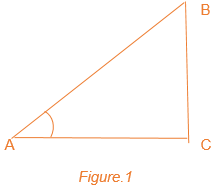From the figure, the following trigonometric ratios can be deducted.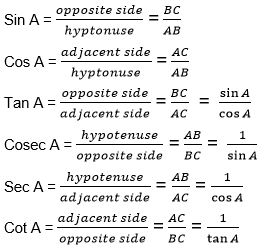Each of these trigonometric ratios can be evaluated at different angles. Some standard angles are given in Table 1 for each of the ratios. From these standard angles, we can extend the evaluation of trigonometric ratios for any angles.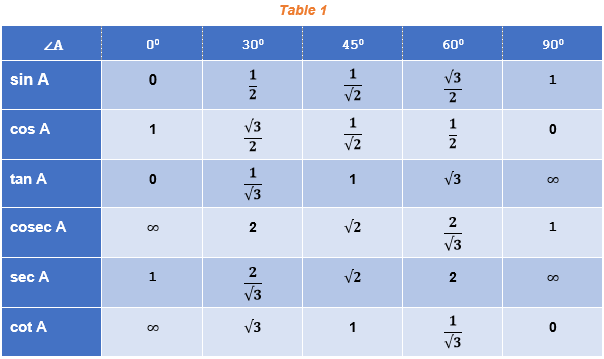Remark : From Table 1 above, you can observe that as ∠ A increases from 0° to 90°, sin A increases from 0 to 1, and cos A decreases from 1 to 0.

Trigonometric ratios of complementary angles

From F igure 1 , it is clear that ∠A + ∠B = 90 °  or ∠B = 90 °   – ∠A. So, consider the value for sine of ∠B, i.e., sin B = sin ( 90 °   – ∠A ) = AC/AB. But AC/AB turns out to be equal to cos A.

In short, we can have sin (90 °   – ∠A) = cos A. Similarly, we can define other trigonometric ratios for complementary angles as follows:

i. sin (90°   – ∠A) = cos A

ii. cos (90°   – ∠A) = sin A

iii. tan (90°   – ∠A) = cot A

iv. cot (90°   – ∠A) = tan A

v. sec (90°   – ∠A) = cosec A

vi. cosec (90°   – ∠A) = sec A.

Identities in Trigonometry

In Figure 1 , it is clear that the right triangle ABC holds Pythagoras property as AB 2 = AC 2 + BC 2

Dividing both sides by AB 2 , we get, 1 = cos 2 A + sin 2 A.

This is a very fundamental identity in trigonometry. Similarly, we can deduce other identities. They are listed below.

sin 2 A + cos 2 A = 1

sec 2 A – tan 2 A = 1

cosec 2 A – cot 2 A = 1

Trigonometric Formulas

There are some important formulas we must keep in mind to solve various trigonometric problems and situations. A few are given below:

cos (2nπ + x) = cos x

sin (2nπ + x) = sin x

cos (-x) = cos x

sin (-x) = – sin x

cos (x + y) = cos x cos y – sin x sin y

cox (x – y) = cos x cos y + sin x sin y

sin (x + y) = sin x cos y + cos x sin y

sin (x – y) = sin x cos y – cos x sin y

sin 3x = 3 sin x – 4 sin 3 x

cos 3x = 4 cos 3 x – 3 cos x

cos x + cos y = 2 cos{(x+y)/2} cos{(x-y)/2}

cos x – cos y = -2 sin{(x+y)/2} sin{(x-y)/2}

sin x + sin y = 2 sin{(x+y)/2} cos{(x-y)/2}

sin x – sin y = 2 cos{(x+y)/2} sin{(x-y)/2}

2 cos x cos y = cos (x + y) + cos (x – y)

-2 sin x sin y = cos (x + y) – cos (x – y)

2 sin x cos y = sin (x + y) + sin (x – y)

2 cos x sin y = sin (x + y) – sin (x – y)

cos(π – x) = -cos x

cos(π + x) = -cos x

sin(π – x) = sin x

sin(π + x) = -sin x

cos(2π – x) = cos x

sin(2π – x) = – sin x

cos (π/2 + x) = – sin x

sin (π/2 + x) = cos x

Radian measure = degree measure x π/180

Degree measure = radian measure x 180/π

## Trigonometric Equations and Its Solutions

Equations involving trigonometric functions of a variable are known as trigonometric equations. Example: cos 2 x + 5 cos x – 7 = 0 , sin 5x + 3 sin 2 x = 6 , etc. The solutions of these equations for a trigonometric function in variable x, where x lies in between 0 ≤ x ≤ 2π, is called the principal solution . If the solution contains the integer ‘ n’ in it, it is called the general solution .

Find the table below, which illustrates the solutions for various trigonometric equations .

Consider the proof for the equation sin x = sin y implies x = nπ + (-1) n y, where n € Z and x and y are any real numbers.

· If sin x = sin y , then sin x – sin y =0 , i.e. 2 cos(x+y)/2 sin(x-y)/2 =0

· It gives cos (x+y)/2 = 0 or (x+y)/2 = (2n + 1) π/2

· Similarly, sin (x-y)/2 = 0 or (x-y)/2 = nπ

· It implies x = (2n+1) π – y ; or x= 2nπ +y

· Hence, x = (2n+1) π + (-1) 2n+1 y ; or x = 2nπ + (-1) 2n y

· Combining these two results, we get x = nπ + (-1) n y, where n ∈ Z

## Video Lessons

Trigonometric equations full chapter in one-shot for jee mains 2023.JEE TrigonometrySolve JEE Trigonometry Problems## Example Problems on Trigonometric Equations

The solved problems given below would help students to co-relate with the formulas covered so far.

Example 1: If f(x) = tan 3x, g(x) = cot (x – 50) and h(x) = cos x, find x given f(x) = g(x). Also, if h(x) = 4/5, find cosec x + tan 3 x.

Solution : If f(x) = g(x), so tan 3x = cot (x – 50)

⇒ cot (90 – 3x) = cot (x-50)

⇒ 90 – 3x = x – 50

For h(x)=cos x and h(x) = 4/5, we have cos x = 4/5.

Therefore, sin x = 3/5, cosec x = 5/3 and tan x = 4/5

Or, cosec x + tan 3 x = (5/3) + (4/5) 3 = 817/375 = 2.178.

Example 2: Find the principal solutions of the equation tan x = – 1/(√3).

Soln : We know that tan(π/6) = 1/(√3)

Since, tan (π – π/6 ) = -tan(π/6) = – 1/(√3)

Further, tan (2π – π/6) = -tan(π/6) = – 1/(√3)

Hence, the principal solutions are tan (π – π/6) = tan (5π/6) and tan (2π – π/6 ) = tan (11π/6).

Example 3: Evaluate the value of sin (11π/12).

sin (11π/12) can be written as sin (2π/3 + π/4)

using formula, sin (x + y) = sin x cos y + cos x sin y

sin (11π/12) = sin (2π/3 + π/4) = sin(2π/3) cos π/4 + cos(2π/3) sin π/4

= (√3)/2 × √2/2 + (-1/2) × √2/2

= √6/4 – (√2)/4

= (√6-√2)/4

Example 4: Evaluate cosec x = 2.

Solution : We know that cosec x = cosec π/6 = 2 or sin x = sin π/6 = 1/2.

Or, x = n π + (-1) n π/6

Example 5: Solve 5 cos 2 y + 2 sin y = 0.

Solution : 5 cos 2 y + 2 sin y = 0

or 5 (1 – sin 2 y) + 2 sin y = 0

or 5 sin 2 y – 2 sin y – 5 =0

i.e., sin y = 1.2 or sin y = -0.8.

Since sin y can not be greater than 1,

sin y = – 0.8 = sin ( π + π/3 )

or sin y = sin 4π/3, and hence, the solution is given by y = n π + (-1) n 4π/3.

Example 6: Find the principal solutions of the equation sin x = (√3)/2.

Solution : We know that, sin π/3 = (√3)/2 and sin 2π/3 = sin (π – π/3 ) = sin π/3 = (√3)/2

Therefore, the principal solutions are x = π/3 and 2π/3.

## Trigonometric Equations – Important Topics## Trigonometric Equations – Rapid RevisionGive the formula of trigonometric identities..

sin 2 x + cos 2 x = 1 sec 2 x – tan 2 x = 1 cosec 2 x – cot 2 x = 1.

## What is the value of tan 60 0 ?

The value of tan 60 0 = √3.

## Give the formula for trigonometric ratios of compound angles for cos (A + B) and cos (A – B).

cos (A + B) = cos A cos B – sin A sin B. cos (A – B) = cos A cos B + sin A sin B.Put your understanding of this concept to test by answering a few MCQs. Click ‘Start Quiz’ to begin!

Select the correct answer and click on the “Finish” button Check your score and answers at the end of the quiz

Visit BYJU’S for all JEE related queries and study materials

Request OTP on Voice Call

Post My Comment• Share Share

Register with byju's & watch live videos.#### IMAGES

1. Solving Trigonometric Equations (Degrees)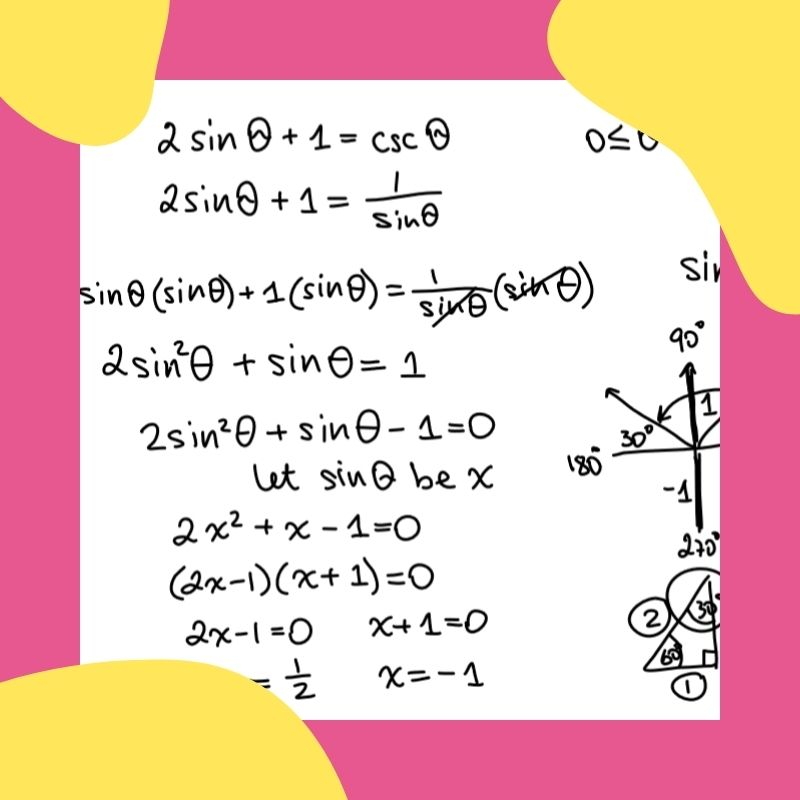2. Solving Trig Equations 1 Video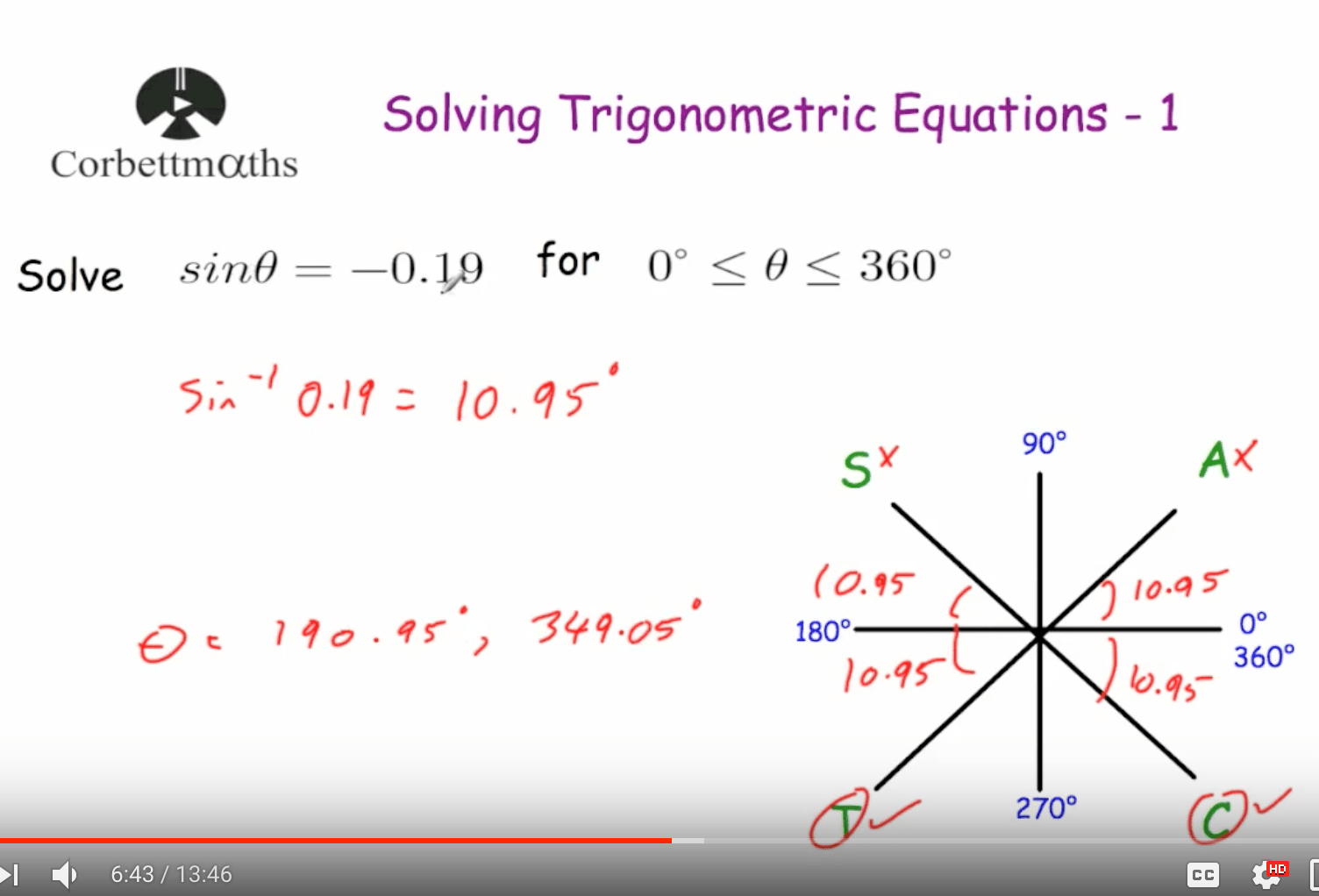3. 5.5B Solving Trig Equations with Factoring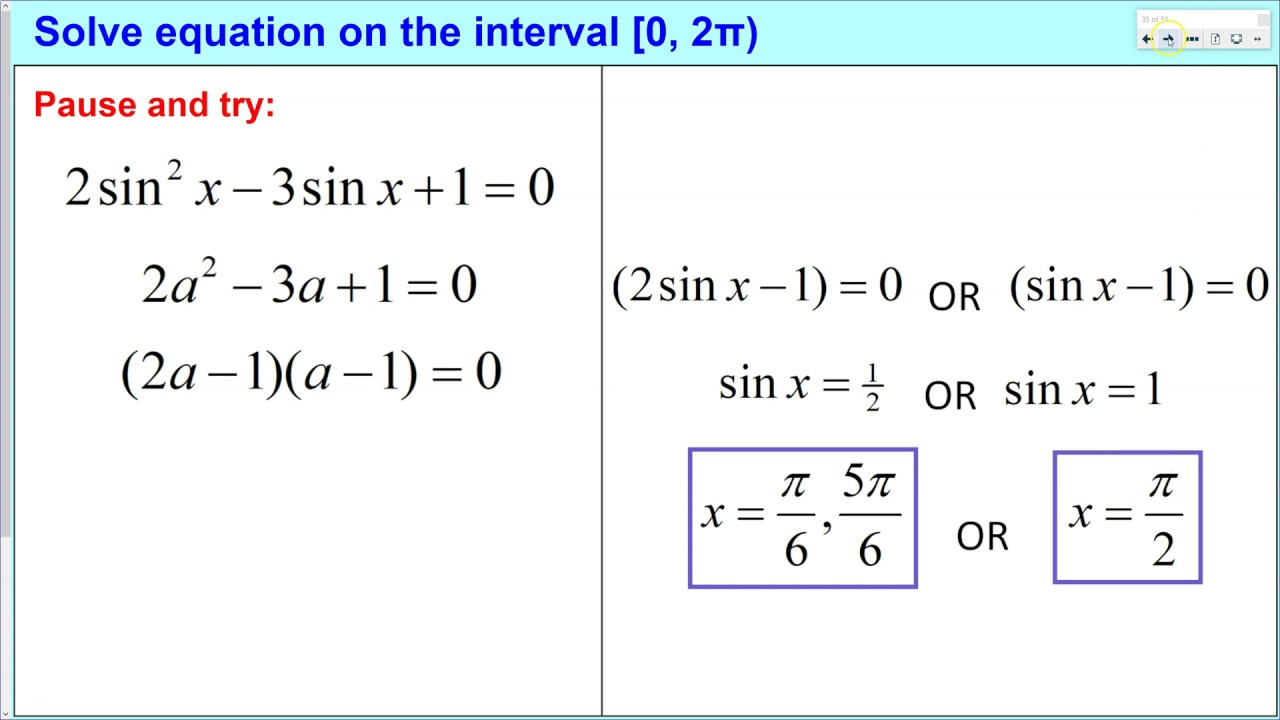4. Solving Trig Equations 2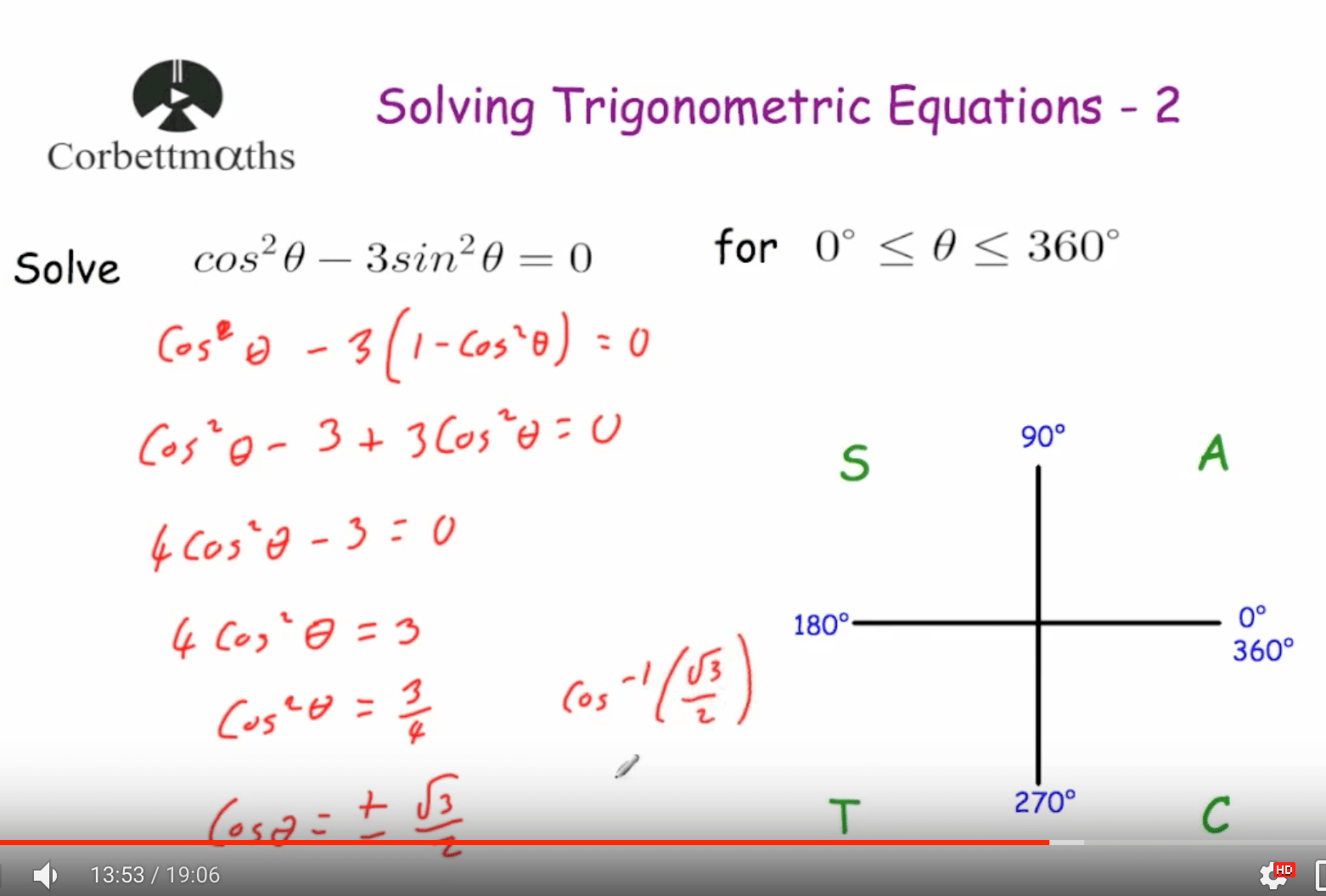5. Solving Trig Equations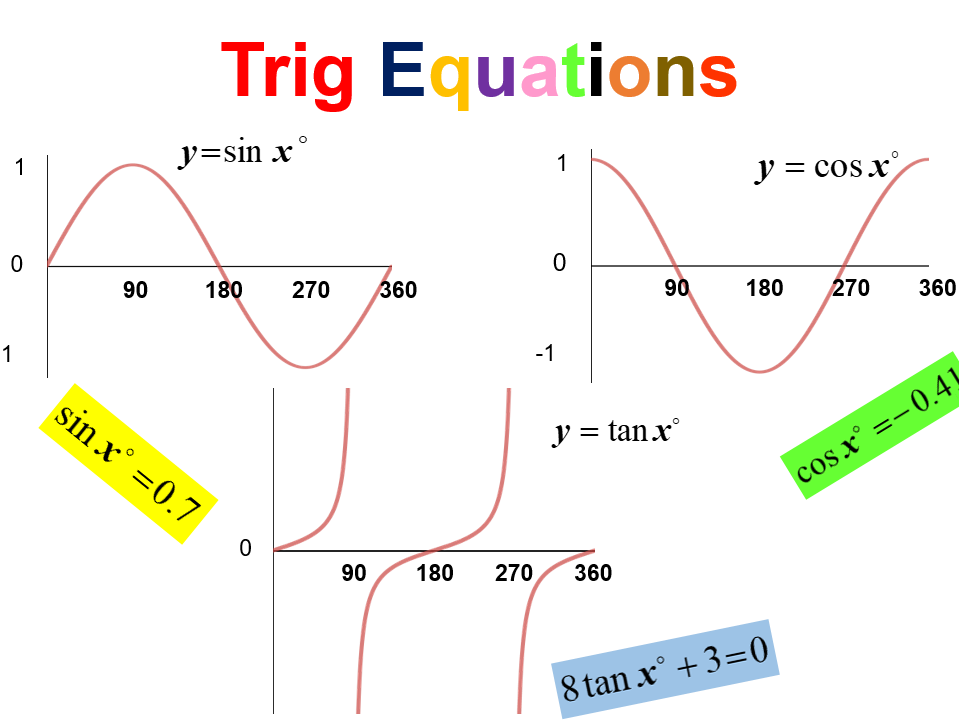6. How To Solve Trigonometric Equations With Multiple Angles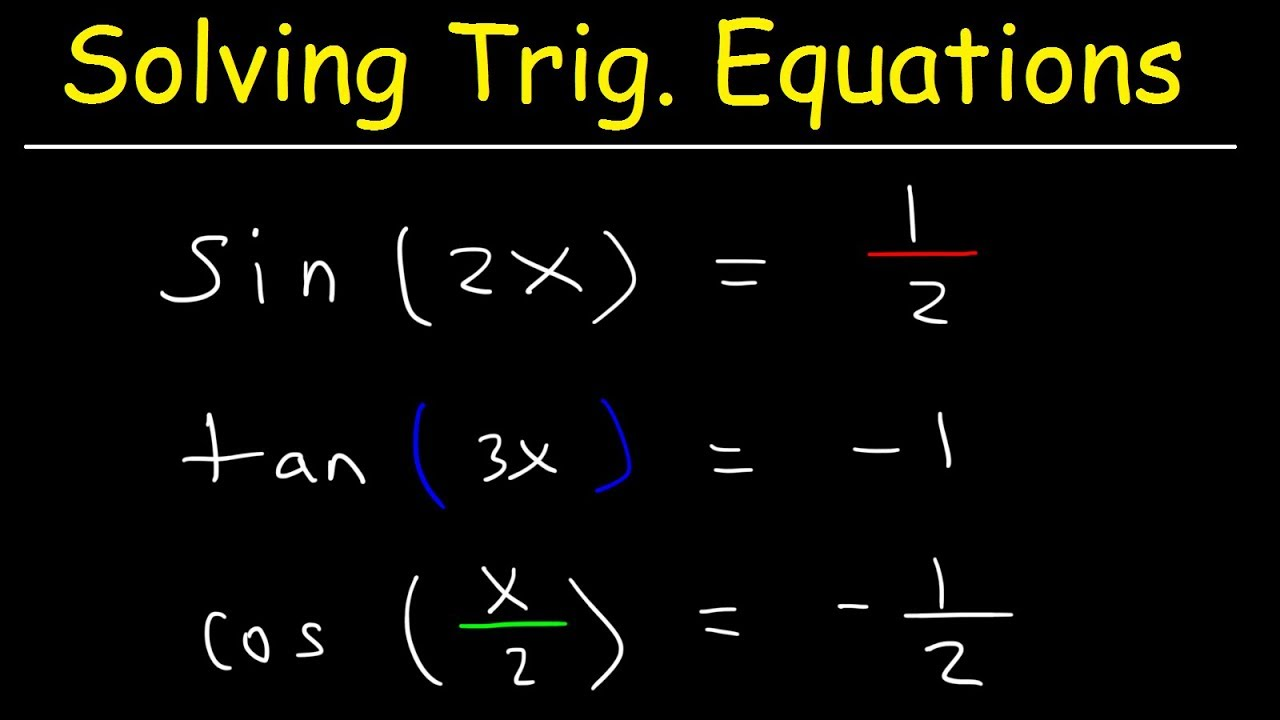#### VIDEO

1. AP Precal 3.10 Day 2 (1-6)

2. 5.3B Solving Trig Equations Using Inverse of sine

3. Solving complex Trig Equation

4. Precalculus Notes 13.5 Solving Trig Equations

5. solving trig equations with a substitute identity

6. solving trig equation part1

1. 3.3: Solving Trigonometric Equations

3.3: Solving Trigonometric Equations

2. 9.1: Solving Trigonometric Equations with Identities

The Pythagorean identities are based on the properties of a right triangle. cos2θ + sin2θ = 1. 1 + cot2θ = csc2θ. 1 + tan2θ = sec2θ. The even-odd identities relate the value of a trigonometric function at a given angle to the value of the function at the opposite angle. tan( − θ) = − tanθ. cot( − θ) = − cotθ.

3. Trigonometric equations and identities

You'll learn how to use trigonometric functions, their inverses, and various identities to solve and check equations and inequalities, and to model and analyze problems involving periodic motion, sound, light, and more. Inverse trigonometric functions Learn Intro to arcsine Intro to arctangent Intro to arccosine

4. Solving Trigonometric Equations

Solving trigonometric equations requires the same techniques as solving algebraic equations. We read the equation from left to right, horizontally, like a sentence. We look for known patterns, factor, find common denominators, and substitute certain expressions with a variable to make solving a more straightforward process.

5. 7.5 Solving Trigonometric Equations

Solving trigonometric equations requires the same techniques as solving algebraic equations. We read the equation from left to right, horizontally, like a sentence. ... The basic rules of algebra apply here, as opposed to rewriting one side of the identity to match the other side. In the next example, we use two identities to simplify the ...

6. How to Solve Trigonometric Equations: 8 Steps (with Pictures)

Know how to solve basic trig equations. There are 4 types of basic trig equations: sin x = a ; cos x = a; tan x = a ; cot x = a; Solving basic trig equations proceeds by studying the various positions of the arc x on the trig circle, and by using trig conversion table (or calculator).To fully know how to solve these basic trig equations, and similar, see book titled :"Trigonometry: Solving ...

7. Trigonometric functions

This topic covers: - Unit circle definition of trig functions - Trig identities - Graphs of sinusoidal & trigonometric functions - Inverse trig functions & solving trig equations - Modeling with trig functions - Parametric functions Introduction to radians Learn Intro to radians Radians & degrees Degrees to radians Radians to degrees

8. Trigonometry

Solving for an angle in a right triangle using the trigonometric ratios Sine and cosine of complementary angles Modeling with right triangles The reciprocal trigonometric ratios Unit 2: Trigonometric functions 0/1900 Mastery points

9. Solving trigonometric equations in degrees

Trigonometric equations can be solved in degrees or radians using CAST and its period to find other solutions within the range, including multiple or compound angles and the wave function. Part...

10. 6.1: Solving Trigonometric Equations

Example 6.1. Solve the equation 2 sin θ + 1 = 0 2 sin θ + 1 = 0. Solution: Isolating sin θ sin θ gives sin θ = − 12 sin θ = − 1 2. Using the sin−1 sin − 1 calculator button in degree mode gives us θ = −30∘ θ = − 30 ∘, which is in QIV. Recall that the reflection of this angle around the y y -axis into QIII also has the ...

11. Introduction to Trigonometry

The general rule is: When we know any 3 of the sides or angles we can find the other 3 (except for the three angles case) See Solving Triangles for more details. Other Functions (Cotangent, Secant, Cosecant) Similar to Sine, Cosine and Tangent, there are three other trigonometric functions which are made by dividing one side by another:

12. Basic trigonometric equations

Trigonometric equations can be solved in degrees or radians using CAST and its period to find other solutions within the range, including multiple or compound angles and the wave function. Part...

13. Solving Trigonometric Equations using Algebraic Methods

To solve a trigonometric equation, we use the rules of algebra to isolate the trigonometric function on one side of the equal sign. Then we use our knowledge of the values of the trigonometric functions to solve for the variable. When you solve a trigonometric equation that involves only one trigonometric expression, begin by isolating the ...

14. Calculus I

Example 1 Solve 2cos(t) = √3 . Show Solution Now, in a calculus class this is not a typical trig equation that we'll be asked to solve. A more typical example is the next one. Example 2 Solve 2cos(t) = √3 on [ − 2π, 2π] . Show Solution So, let's see if you've got all this down. Example 3 Solve 2sin(5x) = − √3 on [ − π, 2π] . Show Solution

15. Trigonometry

Knowing trig identities is one thing, but being able to prove them takes us to another level. In this unit, we'll prove various trigonometric identities and define inverse trigonometric functions, which allow us to solve trigonometric equations.

16. Section 1.5 : Solving Trig Equations with Calculators, Part I

So, in other words, when we are using our calculator to compute an inverse trig function we are really solving a simple trig equation. Having our calculator compute cos−1(3 4) cos − 1 ( 3 4) and hence solve cos(x) = 3 4 cos ( x) = 3 4 gives, x =cos−1( 3 4) =0.7227 x = cos − 1 ( 3 4) = 0.7227. From the previous section we know that there ...

17. Solving Trigonometric Equations By Finding All Solutions

This trigonometry video provides a basic introduction into solving trigonometric equations. it explains how to find all solutions by representing the soluti...

18. Calculus I

Section 1.4 : Solving Trig Equations. Back to Problem List. 1. Without using a calculator find all the solutions to 4sin(3t) = 2 4 sin ( 3 t) = 2. Show All Steps Hide All Steps. Start Solution.

19. 7.1 Solving Trigonometric Equations with Identities

Introduction to Systems of Equations and Inequalities; 9.1 Systems of Linear Equations: Two Variables; 9.2 Systems of Linear Equations: Three Variables; 9.3 Systems of Nonlinear Equations and Inequalities: Two Variables; 9.4 Partial Fractions; 9.5 Matrices and Matrix Operations; 9.6 Solving Systems with Gaussian Elimination; 9.7 Solving Systems with Inverses; 9.8 Solving Systems with Cramer's Rule

20. Solving Trigonometric Equations

Example 1: Solving Trig Equations in Radians. Let's start with a simple example and solve 2 s i n ( θ) = ( 2). The first step is to isolate the trig function: s i n ( θ) = ( 2) / 2.

21. Trigonometric Equations and General Solutions

Equations involving trigonometric functions of a variable are known as trigonometric equations. Example: cos 2 x + 5 cos x - 7 = 0 , sin 5x + 3 sin 2 x = 6 , etc. The solutions of these equations for a trigonometric function in variable x, where x lies in between 0 ≤ x ≤ 2π, is called the principal solution.

22. 3.6: Solving Trigonometric Equations

Math 39: Trigonometry

23. Calculus I

Section 1.4 : Solving Trig Equations. Without using a calculator find the solution (s) to the following equations. If an interval is given find only those solutions that are in the interval. If no interval is given find all solutions to the equation. 4sin(3t) = 2 4 sin. ⁡. ( 3 t) = 2 Solution. 4sin(3t) = 2 4 sin. ⁡.